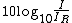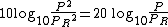# decibel

(redirected from Bel (acoustics))
Also found in: Dictionary, Thesaurus, Medical.

## decibel

decibel (dĕsˈəbĕlˌ, –bəl), abbr. dB, unit used to measure the loudness of sound. It is one tenth of a bel (named for A. G. Bell), but the larger unit is rarely used. The decibel is a measure of sound intensity as a function of power ratio, with the difference in decibels between two sounds being given by dB=10 log10(P1/P2), where P1 and P2 are the power levels of the two sounds. The faintest audible sound, corresponding to a sound pressure of about 0.0002 dyne per sq cm, is arbitrarily assigned a value of 0 dB. The loudest sounds that can be tolerated by the human ear are about 120 dB. The level of normal conversation is about 50 to 60 dB. The decibel is also used to measure certain other quantities, such as power loss in telephone lines.

## Decibel

A logarithmic unit used to express the magnitude of a change in level of power, voltage, current, or sound intensity. A decibel (dB) is 1/10 bel.

In acoustics a step of 1 bel is too large for most uses. It is therefore the practice to express sound intensity in decibels. The level of a sound of intensity I in decibels relative to a reference intensity IR is given by notation (1).

(1)Because sound intensity is proportional to the square of sound pressure P, the level in decibels is given by Eq. (2).
(2)The reference pressure is usually taken as 0.0002 dyne/cm2 or 0.0002 microbar. (The pressure of the Earth's atmosphere at sea level is approximately 1 bar.) See Sound pressure

The neper is similar to the decibel but is based upon natural (napierian) logarithms. One neper is equal to 8.686 dB.

McGraw-Hill Concise Encyclopedia of Physics. © 2002 by The McGraw-Hill Companies, Inc.
The following article is from The Great Soviet Encyclopedia (1979). It might be outdated or ideologically biased.

## Decibel

a fractional unit of the bel (a unit of relative logarithmic value—the common logarithm of the ratio of two synonymous physical quantities, such as energy, power, or sound pressure). The decibel is equal to 0.1 bel. Symbols: Russian, db; international, dB. In practice the decibel is more often used than the bel, the basic unit.

## decibel

[′des·ə‚bel]
(physics)
A unit for describing the ratio of two powers or intensities, or the ratio of a power to a reference power; in the measurement of sound intensity, the pressure of the reference sound is usually taken as 2 × 10-4 dyne per square centimeter; equal to one-tenth bel; if P1 and P2 are two amounts of power, the first is said to be n decibels greater, where n = 10 log10(P1/ P2). Abbreviated dB.
McGraw-Hill Dictionary of Scientific & Technical Terms, 6E, Copyright © 2003 by The McGraw-Hill Companies, Inc.

## decibel

The unit in which the level, 4 of various acoustical quantities is expressed.
McGraw-Hill Dictionary of Architecture and Construction. Copyright © 2003 by McGraw-Hill Companies, Inc.

## decibel

1. a unit for comparing two currents, voltages, or power levels, equal to one tenth of a bel
2. a similar unit for measuring the intensity of a sound. It is equal to ten times the logarithm to the base ten of the ratio of the intensity of the sound to be measured to the intensity of some reference sound, usually the lowest audible note of the same frequency
Collins Discovery Encyclopedia, 1st edition © HarperCollins Publishers 2005

## deciBel

A unit of measurement of the loudness or strength of a signal. One deciBel is considered the smallest difference in sound level that the human ear can discern. Created in the early days of telephony as a way to measure cable and equipment performance and named after Alexander Graham Bell, deciBels (dBs) are a relative measurement derived from two signal levels: a reference input level and an observed output level. A deciBel is the logarithm of the ratio of the two levels. One Bel is when the output signal is 10x that of the input, and one deciBel is 1/10th of a Bel.

Sound Levels
A whisper is about 20 dB. A normal conversation is typically from 60 to 70 dB, and a noisy factory from 90 to 100 dB. Loud thunder is approximately 110 dB, and 120 dB borders on the threshold of pain. In 1883, the volcano on the Indonesian island of Krakatoa erupted. A hundred miles away, the sound level reached more than 170 dB, deafening everyone who survived. See dBm.
```INCREASE IN POWER LEVELS (WATTS)Formula is dB=10*log(P1/P2)DeciBels   Output Signal Strength
3dB           2x
6dB           4x
10dB (1 Bel)  10x
20dB         100x
30dB       1,000x
40db      10,000x

ATTENUATION OF AMPLITUDE (VOLTS or AMPS)Formula is dB=20*log(A1/A2)DeciBels   Output Signal Strength
-3dB        0.707x
-6dB        0.5x
-10dB        0.316x
-20dB        0.1x
-30dB        0.032x
-40db        0.010x
```

Bels and BellsQuite a lot was named after Alexander Graham Bell. Throughout the 20th century, the Bell name was ubiquitous. It will live on with the deciBel.
Copyright © 1981-2019 by The Computer Language Company Inc. All Rights reserved. THIS DEFINITION IS FOR PERSONAL USE ONLY. All other reproduction is strictly prohibited without permission from the publisher.
Site: Follow: Share:
Open / Close